# Samacheer Kalvi 12th Accountancy Solutions Chapter 4 Goodwill in Partnership Accounts

Students can Download Accountancy Chapter 4 Goodwill in Partnership Accounts Questions and Answers, Notes Pdf, Samacheer Kalvi 12th Accountancy Book Solutions Guide Pdf helps you to revise the complete Tamilnadu State Board New Syllabus and score more marks in your examinations.

## Tamilnadu Samacheer Kalvi 12th Accountancy Solutions Chapter 4 Goodwill in Partnership Accounts

### Samacheer Kalvi 12th Accountancy Goodwill in Partnership Accounts Text Book Back Questions and Answers

Question 1.
Which of the following statements is true?
(a) Goodwill is an intangible asset
(b) Goodwill is a current asset
(c) Goodwill is a fictitious asset
(d) Goodwill
(a) Goodwill is an intangible asset

Question 2.
Super profit is the difference between ………………..
(a) Capital employed and average profit
(b) Assets and liabilities
(c) Average profit and normal profit
(d) Current year’s profit and average profit
(c) Average profit and normal profit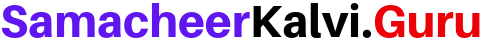Question 3.
The average rate of return of similar concerns is considered as ………………..
(a) Average profit
(b) Normal rate of return
(c) Expected rate of return
(d) None of these
(b) Normal rate of return

Question 4.
Which of the following is true?
(a) Super profit = Total profit / number of years
(b) Super profit = Weighted profit / number of years
(c) Super profit = Average profit – Normal profit
(d) Super profit = Average profit x Years of purchase
(c) Super profit = Average profit – Normal profit

Question 5.
Identify the incorrect pair ………………..
(a) Goodwill under Average profit method – Average profit x Number of years of purchase
(b) Goodwill under Super profit method – Super profit x Number of years of purchase
(c) Goodwill under Annuity method – Average profit x Present value of annuity factor
(d) Goodwill under Weighted average profit method – Weighted average profit x Number of years of purchase
(c) Goodwill under Annuity method – Average profit x Present value of annuity factor

Question 6.
When the average profit is ₹ 25,000 and the normal profit is ₹ 15,000, super profit is ………………..
(a) ₹ 25,000
(b) ₹ 5,000
(c) ₹ 10,000
(d) ₹ 15,000
(c) ₹ 10,000Question 7.
Book profit of 2017 is ₹ 35,000; non – recurring income included in the profit is ₹ 1,000 and abnormal loss charged in the year 2017 was ₹ 2,000, then the adjusted profit is ………………..
(a) ₹ 36,000
(b) ₹ 35,000
(c) ₹ 38,000
(d) ₹ 34,000
(a) ₹ 36,000

Question 8.
The total capitalised value of a business is ₹ 1,00,000; assets are ₹ 1,50,000 and liabilities are ₹ 80,000. The value of goodwill as per the capitalisation method will be ………………..
(a) ₹ 40,000
(b) ₹ 70,000
(c) ₹ 1,00,000
(d) ₹ 30,000
(d) ₹ 30,000

Question 1.
What is goodwill?
Goodwill is the good name or reputation of the business which brings benefit to the business. It enables the business to earn more profit. It is the present value of a firm’s future excess earnings.

Question 2.
What is acquired goodwill?
Goodwill acquired by making payment in cash or kind is called acquired or purchased goodwill. When a firm purchases an existing business, the price paid for purchase of such business may exceed the net assets (Assets – Liabilities) of the business acquired.Question 3.
What is super profit?
Super profit is the excess of average profit over the normal profit of a business.
Super profit = Average profit – Normal profit.
Average profit is calculated by dividing the total of adjusted actual profit of certain number of years by the total number of such years. Normal profit is the profit earned by the similar business firms under normal conditions.
Normal profit = Capital employed x Normal rate of return Capital employed = Fixed assets + Current assets – Current liabilities

Question 4.
What is normal rate of return?
It is the rate at which profit is earned by similar business entities in the industry under normal circumstances.

Question 5.
State any two circumstances under which goodwill of a partnership firm is valued?

1. When there is a change in the profit sharing ratio.
2. When a new partner is admitted into a firm.
3. When an existing partner retires from the firm or when a partner dies.
4. When a partnership firm is dissolved.

Question 1.
State any six factors determining goodwill.

1. Profitability of the firm.
2. Favourable location of the business enterprise.
3. Good quality of goods or services offered.
4. Tenure of the business enterprise.
5. Efficiency of management.
6. Degree of competition.
7. Other factors.

Question 2.
How is goodwill calculated under the super profits method?
1. Purchase of super profit method: Goodwill is calculated by multiplying the super profit by a certain number of years of purchase.
Goodwill = super profit x No. of years of purchase

2. Annuity method: Value of goodwill is calculated by multiplying the super profit with the present value of annuity.
Goodwill = Super profit x Present value annuity factor

3. Capitalisation of super profit method: Goodwill =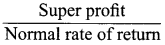x 100

Question 3.
How is the value of goodwill calculated under the capitalisation method?
Capitalisation method:
Under Capitalisation method, goodwill is the excess of capitalised value of average profit of the business over the actual capital employed in the business.
Goodwill = Total capitalised value of the business – Actual capital employed
The total capitalised value of the business is calculated by capitalising the average profits on the basis of the normal rate of return.
Capitalised value of the business =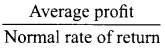x 100
Actual capital employed = Fixed assets (excluding goodwill) + Current assets – Current liabilitiesQuestion 4.
Compute average profit from the following information.
Calculation of Average profit:
2016 – ₹ 8,000; 2017 – ₹ 10,000; 2018 – ₹ 9,000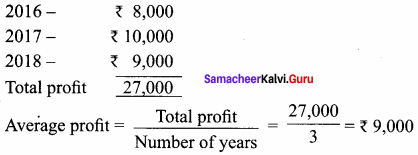Valuation of goodwill = ₹ 9,000

Question 5.
Calculate the value of goodwill at 2 years purchase of average profit when average profit is ₹ 15,000.
Goodwill: ₹ 30,000

IV. Exercises

Simple average profit method:
Question 1.
The following are the profits of a firm in the last five years: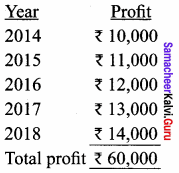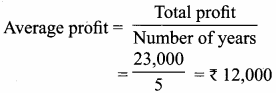Valuation of goodwill = Average profit x No. of years purchase
= ₹ 12,000 x 2 years
= ₹ 24,000

Question 2.
From the following information, calculate the value of goodwill on the basis of 3 years purchase of average profits of last four years.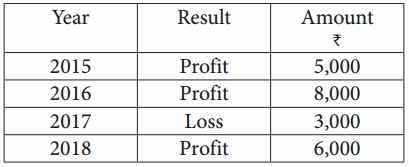Calculation of goodwill: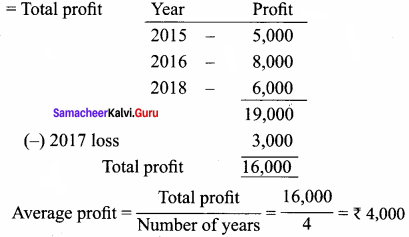Valuation of goodwill = Average profit x No. of years purchase = ₹ 4,000 x 3
= ₹ 12,000

Question 3.
From the following information relating to a partnership firm, find out the value of its goodwill based on 3 years purchase of average profits of the last 4 years:

1. Profits of the years 2015, 2016, 2017 and 2018 are ₹ 10, 000, ₹ 12, 500, ₹ 12, 000 and ₹ 11, 500, respectively.
2. The business was looked after by a partner and his fair remuneration amounts to ₹ 1, 500 per year. This amount was not considered in the calculation of the above profits.

Valuation of goodwill
Calculation of average profit Year Profit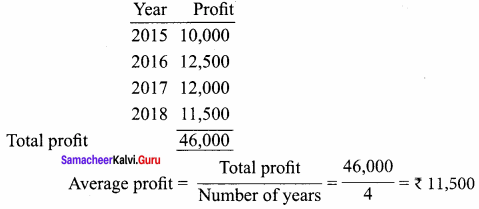(-) Remuneration = ₹ 1,500
Net average profit = ₹ 10,000
Valuation of goodwill = Average profit x No. of years purchase
= ₹ 10,000 x 3
= ₹ 30,000Question 4.
From the following information relating to Sridevi enterprises, calculate the value of goodwill on the basis of 4 years purchase of the average profits of 3 years.

1. Profits for the years ending 31st December 2016, 2017 and 2018 were ₹ 1,75,000, ₹ 1,50,000 and ₹ 2,00,000, respectively.
2. A non – recurring income of ₹ 45,000 is included in the profits of the year 2016.
3. The closing stock of the year 2017 was overvalued by ₹ 30,000.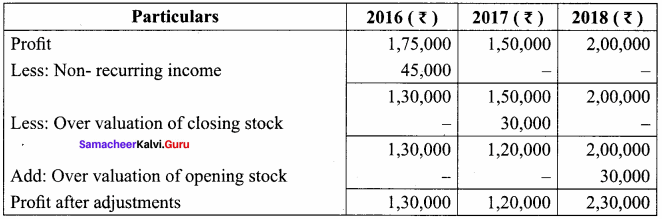Average profit = <Average profit = $$\frac { 4, 80, 000 }{ 3 }$$ = ₹ 1,60,000
Goodwill = Average profit x No. of year purchase = ₹ 1,60,000 x 4
= ₹ 64,00,000

Question 5.
The following particulars are available in respect of the business carried on by a partnership firm:

1. Profits earned: 2016: ₹ 25,000; 2017: ₹ 23,000 and 2018: ₹ 26,000.
2. Profit of 2016 includes a non – recurring income of ₹ 2,500.
3. Profit of 2017 is reduced by ₹ 3,500 due to stock destroyed by fire.
4. The stock was not insured. But, it is decided to insure the stock in future. The insurance premium is estimated to be ₹ 250 per annum.

You are required to calculate the value of goodwill of the firm on the basis of 2 years purchase of average profits of the last three years.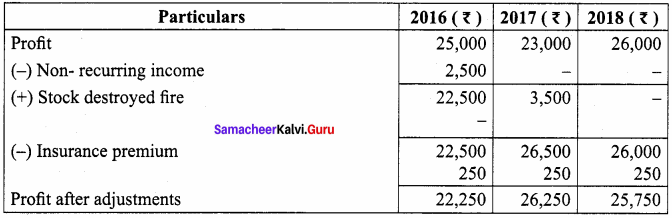Total profit = 22,250 + 26, 250 + 25, 750 = ₹ 74, 250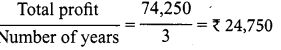Valuation of goodwill = Average profit x No.of year purchase
= ₹ 24, 750 x 2 years = ₹ 49, 500

Weighted average profit method:
Question 6.
Find out the value of goodwill at three years purchase of weighted average profit of last four years.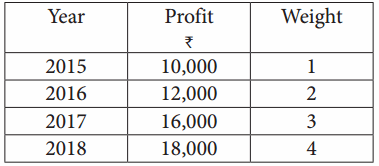Calculation of weighted average profitGoodwill = Weighted average profit x No. of years purchase
= ₹ 15,400 x 3 = ₹ 46,200

Purchase of super profit method:
Question 7.
From the following details, calculate the value of goodwill at 2 years purchase of super profit:

1. Total assets of a firm are ₹ 5,00,000
2. The liabilities of the firm are ₹ 2,00,000
3. Normal rate of return in this class of business is 12.5 %.
4. Average profit of the firm is ₹ 60,000.

Capital employed = fixed assets + current assets – current liabilities
= 5, 00, 000 – 2, 00, 000 = 3, 00, 000
Normal profit = Capital employed x Normal rate of return
= 3,00,000 x $$\frac { 12.5 }{ 100 }$$ = 3, 75, 000
Super profit = Average profit – Normal profit
= 60, 000 – 37, 500 = 22, 500
Goodwill = Super profit x Number of years of purchase
= ₹ 22,500 x 2
= ₹ 45,000Question 8.
A partnership firm earned net profits during the last three years as follows:
2016: ₹ 20,000; 2017: ₹ 17,000 and 2018: ₹ 23,000
The capital investment of the firm throughout the above mentioned period has been ₹ 80,000. Having regard to the risk involved, 15% is considered to be a fair return on capital employed in the business. Calculate the value of goodwill on the basis of 2 years purchase of super profit.
Calculation of average profit Year Profit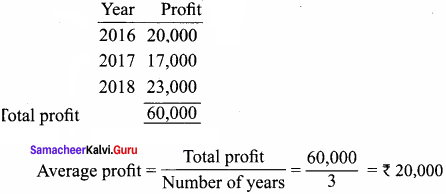Normal profit = Capital employed x Normal rate of return
= 80,000 x $$\frac { 15 }{ 100 }$$ = 12,000
Super profit = 8,000
Valuation of goodwill = Super profit x No. of years purchase
= ₹ 8,000 x 2
= ₹ 16,000

Annuity method:
Question 9.
From the following information, calculate the value of goodwill under annuity method:

1. Average profit – ₹ 14,000
2. Normal profit – ₹ 4,000
3. Normal rate of return – 15%
4. Years of purchase of goodwill – 5

Present value of ₹ 1 for 5 years at 15% per annum as per the annuity table is 3.352
Super profit = Average profit – Normal profit
= 14, 000 – ₹ 4, 000 = ₹ 10, 000
Goodwill = Super profit x Present value of annuity factor
= ₹ 10,000 x 3.352 = ₹ 33, 520Capitalisation of super profit method:
Question 10.
Find out the value of goodwill by capitalising super profits:

1. Normal Rate of Return 10%
2. Profits for the last four years are ₹ 30,000, ₹ 40,000, ₹ 50,000 and ₹ 45,000.
3. Anon – recurring income of ₹ 3,000 is included in the above mentioned profit of ₹ 30,000.
4. Average capital employed is ₹ 3,00,000.Average profit =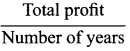= $$\frac { 1, 62, 000 }{ 4 }$$
= ₹ 40,500
Normal profit = Capital employed x Normal rate of return
= 3,00,00 x $$\frac { 10 }{ 100 }$$ = 30,000
Super profit = 10,500
Capitalisation super profit method =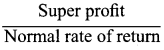x 100
= $$\frac { 10, 500 }{ 10 }$$ = ₹ 1, 05, 000

Capitalisation method:
Question 11.
From the following information, find out the value of goodwill by capitalisation method :

1. Average profit ₹ 20, 000
2. Normal rate of return 10%
3. Tangible assets of the firm ₹ 2, 20, 000
4. Liabilities of the firm ₹ 70, 000

Capital Assets – Liabilities
= 2, 20, 000 – 70, 000 = ₹ 1, 50, 000
Capitalised value of business =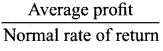x 100
= $$\frac { 20, 000 }{ 10 }$$ x 100 = ₹ 2, 00, 000
Value of goodwill = Total capitalised average profit – Capital employed
= 2, 00, 000 – 1, 50, 000
= ₹ 50,000

### Samacheer Kalvi 12th Accountancy Goodwill in Partnership Accounts Additional Questions and Answers

Question 1.
Goodwill is valued under ………………
(a) Average profit method
(b) Super profit method
(c) Capitalisation method
(d) All of these
(d) All of these

Question 2.
Average profit method can be further divided ………………
(a) Simple average profit
(b) Weighted average profit
(c) Both (a) and (b)
(d) None of these
(c) Both (a) and (b)

Question 3.
Super profit is
(a) Average profit – Normal profit
(b) Normal profit – Average profit
(c) Both (a) and (b)
(d) None of these
(a) Average profit – Normal profitQuestion 4.
Kinds of goodwill ………………
(a) Purchased goodwill
(b) Self – generated goodwill
(c) None of these
(d) Both (a) and (b)
(d) Both (a) and (b)

Question 5.
Nature of goodwill is ………………
(b) Profit of firm
(c) total asset of firm
(d) None of these

Question 6.
Factor of goodwill is ………………
(b) Efficient management
(c) Nature of the goods
(d) All of these
(b) Efficient management

Question 7.
Arrange the following in ascending or descending order:
1. Super profit = Weighted average profit x No. of years purchase
2. Capitalistion method =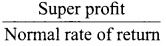x 100
3. Weighted Average method = Average profit – Normal profit
4. Capitalisation super profit = Total profit/No. of years purchase
(a) 1, 2, 3, 4
(b) 2, 4, 1, 2
(c) 3, 4, 1, 2
(d) 4, 3, 2, 1
(c) 3, 4, 1, 2

Question 8.
Goodwill of the firm on the basis of 2 years purchase of average profit of the last 3 years is ₹ 25,000. Find average profit ………………
(a) ₹ 50,000
(b) ₹ 25,000
(c) ₹ 10,000
(d) ₹ 12,500
(a) ₹ 50,000Question 9.
Calculate the value of goodwill at 3 years purchase when capital employed is ₹ 2,50,000. Average profit ₹ 30,000 and normal rate of return is 10% ………………
(a) ₹ 3,000
(b) ₹ 25,000
(c) ₹ 30,000
(d) ₹ 15,000
(d) ₹ 15,000

Question 10.
(a) + past expenses not required in the future
(b) – past revenue not likely to be eached in the future
(c) + additional income expected in the future
(d) All of these
(d) All of these

II. Fill in the blanks:

Question 11.
Goodwill brought in cash by new partner is divided among the old partner by debiting is ________ A/c and crediting ________ A/c.
Goodwill; capital.

Question 12.
Calculation of goodwill under simple average profit method is ________
Total profit =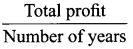Question 13.
Super profit method normal profit is ________
Normal profit = Capital employed x Normal rate of return

Question 14.
Under average profit method ________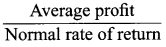x 100

Question 15.
Super profit = ________
Average profit – Normal profit

Question 1.
What are the nature of goodwill?
The nature of the goodwill can be described as follows:

1. Goodwill is an tangible fixed asset. It is tangible because it has no physical existence. It cannot be seen or touched.
2. It has a definite value depending on the profitability of the business enterprise.
3. It cannot be separated from the business.
4. It helps in earning more profit and attracts more customers.
5. It can be purchased or sold only when the business is purchased or sold in full or in part.

Question 2.
What is average profit method?
Under this method, goodwill is calculated as certain years of purchase of average profits of the past years. The number of years of purchase is generally determined on the basis of the average period a new business will take in order to bring it to the current state of the existing business.Question 3.
What is meant by simple average profit method?
Goodwill is calculated by multiplying the average profit by a certain number of years of purchase. Simple average profit is calculated by adding the adjusted profits of certain number of years by dividing the total number of such years.
Goodwill = Average profit x Number of years purchase Total profit.
Average profit =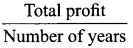Question 4.
Weighted average profit method.
Goodwill is calculated by multiplying the weighted average profit by a certain number of years of purchase.
Goodwill = Weighted average profit x Number of years purchase
In this method, weights are assigned to each year’s profit. Weighted profit is ascertained by multiplying the weights assigned with the respective year’s profit.
Weighted average profit =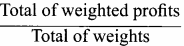Question 5.
What is meant by annuity method of valuation of goodwill?
Value of goodwill is calculated by multiplying the super profit with the present value of annuity.
Goodwill = Super profit x Present value annuity factor
Present value annuity factor is the present value of annuity of rupee one at a given time. It can be found out from annuity table or by using formula.

Question 6.
What is annuity factor?
Annuity refers to series of uniform cash flows at regular intervals. The table value gives the present value of annuity of rupee one received at the end of every year for a specified number of years.where, i = interest rate ; n = estimated number of years

Question 7.
What is capitalisation of super profit method?
Under this method, value of goodwill is calculated by capitalising the super profit at normal rate of return, that is, goodwill is the capitalised value of super profit.
Goodwill =x 100

IV. Exercise

Question 1.
Goodwill is to be valued at three years purchase of five years average profits. The profit for the last five years were 2010 – ₹ 4,200; 2011 — ₹ 4,500; 2012 – ₹ 4,700; 2013 – ₹ 4,600; and 2014 – ₹ 5,000.
Solution:
Calculation of average profit: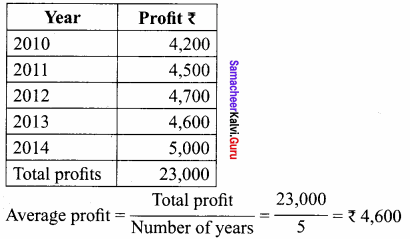Calculation of goodwill = Average profit x No. of years purchase
= ₹ 4,600 x 3 = ₹ 13,800

Question 2.
A firm’s profit for the last 5 years were ₹ 20,000, ₹ 30,000, ₹ 40,000. ₹ 50,000, and ₹ 60,000. Calculated the value of firm’s goodwill on the basis of three years purchase of weighted average profit after using weight of 1, 2, 3, 4, 5 respectively.
Solution:Goodwill = Weighted avg. profit x Number of years purchase = 46,667 x 3 = ₹ 1,40,000 (appr.)

Question 3.
A business has earned average profit ₹ 1,00,000 during the last few years and the normal rate of return in similar business is 10%. Find out the value of goodwill.

1. Capitalisation of super profit method.
2. Super profit method of the goodwill is valued at 3 years purchase of super profit. The assets of the business were ₹ 10,00,000 and liabilities of ₹ 1,80,000.

Solution:
1. Capital employed = Assets – libilities
= 10,00,000 – 1,80,000
= ₹ 8,20,000
Normal profit = Capital employed x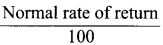8,20,000 = 1 x $$\frac { 10 }{ 100 }$$ = 82,000
Super profit = Average profit – Normal profit
= ₹ 1,00,000 – ₹ 82,000 = ₹ 18,000

2. Capitalisation method Super profit
Goodwill =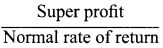x 100
= $$\frac { 18, 000 }{ 10 }$$ = ₹ 1, 80,000
as per super profit method Goodwill = super profit x No. of years purchase
= ₹ 18000 x 3 = ₹ 54,000

Question 4.
The average profit earned by the firm is Z 80,000 which includes under valuation of stock an average basis the capital invested in the business and normal rate of return is 8%. Calculate the goodwill of the firm on the basis of times the super profit.
Solution:
Average profit – ₹ 80,000
(A) Under value of stock – ₹ 8,000
Actual Average profit – ₹ 88,000
Normal profit = Capital investment x Normal rate of return
= ₹ 88,000 – ₹ 64,000
= ₹ 24,000
Goodwill = Super profit x 7
= ₹ 24,000 x 7
= ₹ 1,68,000

Question 5.
Capital investment is ₹ 5,00,000; firms profit ₹ 1,50,000 Assuming that normal rate of return is 20%. Calculate the goodwill

1. Capitalisation method.
2. Super profit method if the goodwill is valued @ 2 years purchase

Solution:
1. Capitalisation of average profit method.
Goodwill =x 100
= $$\frac { 1,50,000 }{ 20 }$$ = ₹ 7,50,000
Goodwill = Total capital employed – capital employed
= ₹ 7,50,000 – ₹ 5,00,000 = ₹ 2,50,000

2. Super profit method
Normal profit = Capital employed x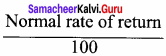Normal profit = ₹ 5,00,000 x $$\frac { 20 }{ 100 }$$ = ₹ 1,00,000
Super profit = Average profit – Normal profit
= ₹ 1,50,000 – 1,00,000
= ₹ 50,000
Value of Goodwill = Super profit x No. of years purchase
= ₹ 50,000 x 2
= ₹ 1,00,000Question 6.
Calculate the value of goodwill of the firm of 2 partners
(a) At the 3 years purchase of average profits
(b) At 3 years purchase of super profits
(c) On the basis of capitalisation of super profits
(d) On the basis of capitalisation of average profits

(i) Average capital employed ₹ 7,00,000
(ii) Net trading results of the firm 2014 – ₹ 1,47,600 2015 – Loss ₹ 1,48,100. Profit for 2016 – ₹ 4,48,700
(iii) Rate of interest on capital @ 18%
(iv) Remunuration ₹ 500/- per month
Solution:
Calculation of Average profit and super profit
Total profit = ₹ 1,47,600 – ₹ 1,48,100 + ₹ 4,48,700 = ₹ 4,48,200
Average profit == ₹ 1,49,400
Less: Remunration of 2 partner (2 x 500 x 1/2) $$\frac { 12,000 }{ 1,37,400 }$$
Less: Normal profit = Capital employed x= 7,00,000 x $$\frac { 18 }{ 100 }$$ = ₹ 1,26,000
Super profit = ₹ 11,400

(a) Average profit – Average profit x No. of year purchase
= ₹ 1,37,400 x 3 = ₹ 4,12,200

(b) Super profit – Super profit x No. of year purchase
= ₹ 11,400 x 3 = ₹ 34,200

(c) Capitalisation of super profit –x 100
= $$\frac { 11,400 }{ 18 }$$ x 100
= ₹ 63,333

(d) Capitalisation of average profit =x 100
= $$\frac { 1,37,400 }{ 100 }$$ x 100
= ₹ 7,63,333
(-) Actual capital employed = ₹ 7,00,000
Goodwill = ₹ 63,333

Scroll to Top